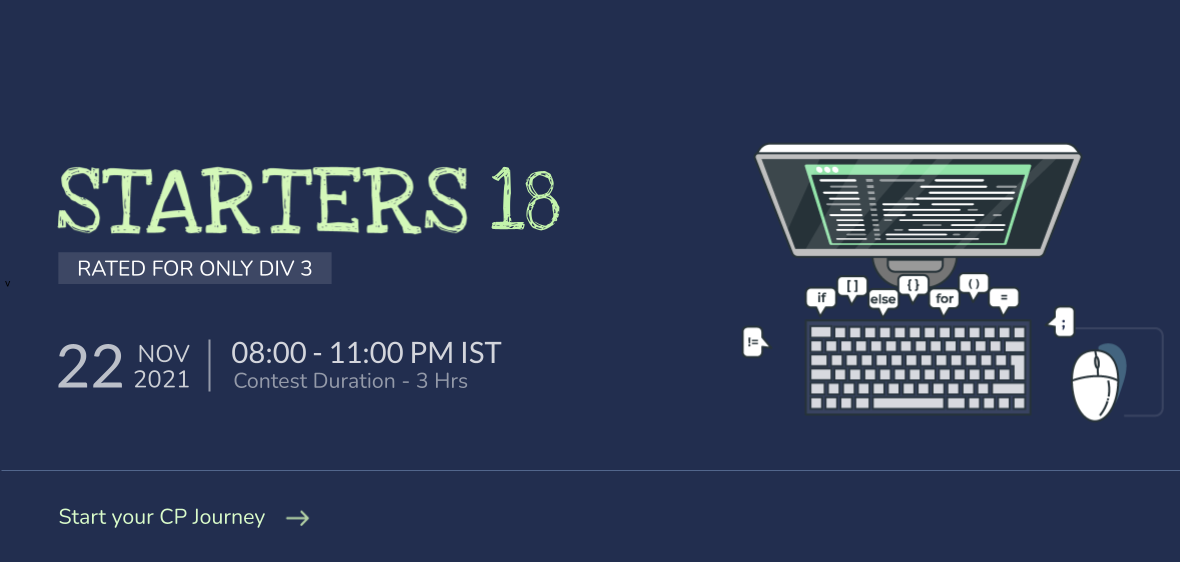# Expectations on a Permutation solution codechef

Contents

## Expectations on a Permutation solution codechef

A permutation of length NN is an array of NN integers A1,A2,,ANA1,A2,…,AN such that every integer from 11 to NN appears in it exactly once.

We define a function over a permutation as follows: F(A)=(A1A2)+(A2A3)++(AN2AN1)+(AN1AN)F(A)=(A1∗A2)+(A2∗A3)+⋯+(AN−2∗AN−1)+(AN−1∗AN)

You are given an integer NN. What is the expected value of the function FF over all possible permutations of length NN?

The expected value of the function can be represented as a fraction of the form PQPQ. You are required to print PQ1(mod1000000007)P⋅Q−1(mod1000000007).### Expectations on a Permutation solution codechef Input Format

• The first line of the input contains a single integer TT denoting the number of test cases. The description of TT test cases follows.
• The first and only line of each test case contains a single integer NN.

### Output Format

For each test case, output on a single line the expected value of the function modulo 10000000071000000007.

• 1T1051≤T≤105
• 2N1092≤N≤109

### Sample Input 1

2
2
3


### Sample Output 1

2
333333343


### Explanation Expectations on a Permutation solution codechef

• Test Case 11: There are 22 possible permutations: A={1,2}A={1,2} with F(A)=2F(A)=2 and A={2,1}A={2,1} with F(A)=2F(A)=2. Hence the expected value of the function is F(A)=122+122=2F(A)=12∗2+12∗2=2.

• Test Case 22: There are 66 possible permutations, with the value of function as {5,5,8,8,9,9}{5,5,8,8,9,9}. Hence the expected value of the function is F(A)=5+5+8+8+9+96=223F(A)=5+5+8+8+9+96=223. The required output will be 333333343333333343, since 333333343322(mod1000000007)333333343⋅3≡22(mod1000000007).